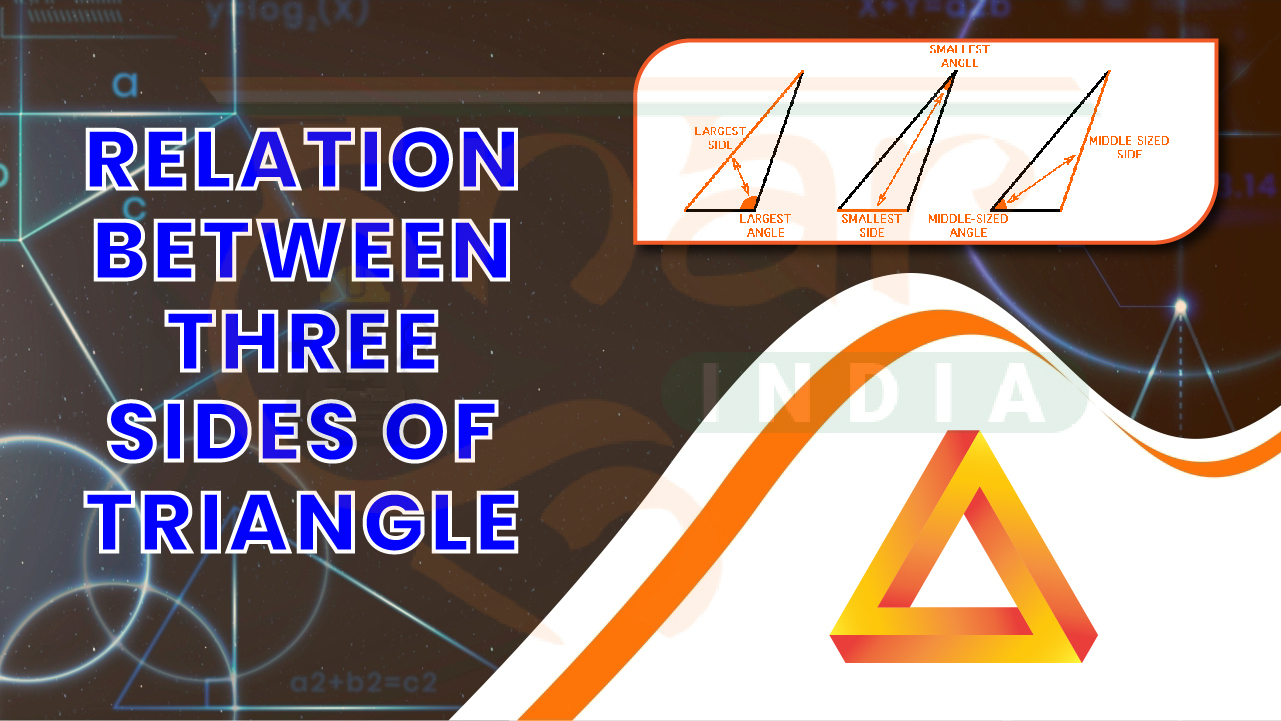×
##### Blog
Relation between 3 sides of Triangle

# Relation between 3 sides of Triangle

#### Tags# Relation between 3 sides of Triangle

In a triangle, the relationship between the lengths of its three sides can provide valuable information about the triangle's properties. Here are some of the key relationships:

1. Triangle Inequality Theorem: According to the Triangle Inequality Theorem, the sum of the lengths of any two sides of a triangle must be greater than the length of the third side. Mathematically, if a, b, and c represent the lengths of the sides of a triangle, then: a + b > c b + c > a a + c > b

2. Equilateral Triangle: In an equilateral triangle, all three sides are equal in length. If "s" represents the length of each side, then: a = b = c = s

3. Isosceles Triangle: In an isosceles triangle, two sides have equal lengths. If "a" represents the length of the two equal sides and "b" represents the length of the remaining side, then: a = b

4. Pythagorean Theorem: In a right triangle, the square of the length of the hypotenuse (the side opposite the right angle) is equal to the sum of the squares of the lengths of the other two sides. If "a" and "b" represent the lengths of the legs, and "c" represents the length of the hypotenuse, then: a^2 + b^2 = c^2

These are some of the fundamental relationships between the lengths of the sides in a triangle. By analyzing the side lengths, mathematicians and scientists can determine the type of triangle, verify its properties, and solve various geometric problems.

Attempt our SSC GD Free Mock Test: https://hunarindia.org.in/testdetail/ssc4-ssc-gd-constable

Attempt our IBPS Clerk Mains Free Mock Test: https://hunarindia.org.in/testdetail/ibps-bank-ibps-clerk-mainsRelation between 3 sides of Triangle.pdf
##### BASIC TERMINOLOGIES OF ACCOUNTANCY

We don't support landscape mode yet. Please go back to Portrait mode for the best experience.

# Hunar India

Typically replies within a day

Hi there